# Cutting Up Space Using n Planes

As the capstone of this series on counting, lets look at something that’s a little harder to count by drawing: What is the maximum number of regions into which all of 3-dimensional space can be divided by n planes? We’ll look at two significantly different perspectives.

## Working up through the dimensions

The first question is from 1998:

Spaces Formed by Intersecting Planes

Do you know of a proof that would be used to show how many subspaces can be formed by the intersecting of five planes in space? This should be the largest number, of course. Pascal's triangle and other patterns can lead to the conclusion that there is 1 space divided by 0 planes, 2 spaces divided by 1 plane, 4 spaces divided by 2 planes, 8 spaces divided by 3, 15 spaces divided by 4, and 26 spaces divided by 26 planes. But is there a proof?

The numbers given here are correct, as we’ll see; it isn’t clear how Kirste is using Pascal’s triangle (the numbers are not obviously present there), so we can’t be sure how close she came to a proof.

Doctor Tom answered, working step by step up to this three-dimensional problem:

Hi Kirste,

There's a nice way to look at this by looking at the problem in lower dimensions.

In the easiest case, 1 dimension, suppose you have a line and you ask how many regions it can be divided into by n points. That's easy: each point divides one of the existing segments into 2, so each new point adds 1, so the total is n+1. (When n is zero, there's one line; adding a point makes 2 half-lines, and so on.)

So in the one-dimensional case, the formula is $$r(n) = n+1$$

We start with 1 region, and add 1 more for each point (new points are in blue here):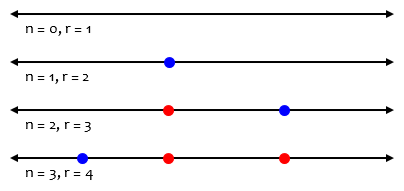Now look at 2 dimensions - a plane divided up by n lines. By messing around, you can see that for n = 0, 1, 2, 3, the answers are: 1, 2, 4, 7 regions, right?  Well, suppose you've worked it out for some number n of lines, and you add the next line. In general, it will hit all n lines, so if you look at the intersections of the old lines with your new one, it gets hit n times, right? So the new line is divided into n+1 segments. (We just worked this out in the previous paragraph).

So each of those n+1 segments will divide an existing region into two regions, so there will be n+1 new regions created, so you can work out the number of 2-D regions you get with n lines:

1 + 1 + 2 + ... + (n+1)

(The initial "1" is because there's already one region when you start.) So the values from the formula above are:

lines   regions
0       1 = 1
1       1+1 = 2
2       1+1+2 = 4
3       1+1+2+3 = 7
4       1+1+2+3+4 = 11
5       1+1+2+3+4+5 = 16
...

This is a quick version of what we saw two weeks ago about cutting a circle with n lines, but now we’re seeing it as an extension of a pattern going from one dimension to the next. Each (n + 1st) line we draw cuts each previous line, so that it is divided by n points, and therefore is divided into n + 1 parts, and adds n + 1 regions in the plane (new lines and points are in blue here):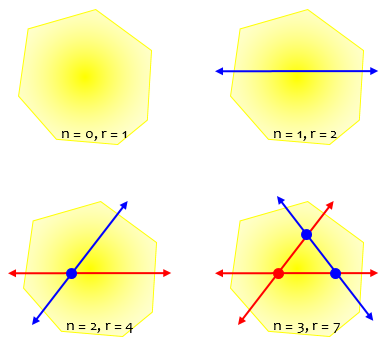For example, that third line adds 3 regions, one for each part into which it is itself divided. when we get to n lines, we have started with 1 region, then added k regions for the kth line, for a total of $$1 + 1 + 2 + 3 + \dots + n$$. We’ll turn this into a formula soon.

Now go to three dimensions. Assume you know the answer for n planes, and you want the answer for n+1. Well, the (n+1)st plane will hit all the n planes in one line each, so that plane is hacked into the number of regions that n lines will create, which we just worked out as the sum above.

Each of those plane regions will divide a volumetric region into 2 pieces, so the answers for 3 dimensions are:

planes   regions
0        1 = 1
1        1+1 = 2
2        1+1+2 = 4
3        1+1+2+4 = 8
4        1+1+2+4+7 = 15
5        1+1+2+4+7+11 = 26
6        1+1+2+4+7+11+16 = 42
...

Each (n + 1st) plane cuts each previous plane in a line, so that it is divided by n lines, and therefore is divided into the number of parts from the previous sequence, thus adding that many new regions to space (new planes and the lines that divide them are in blue here):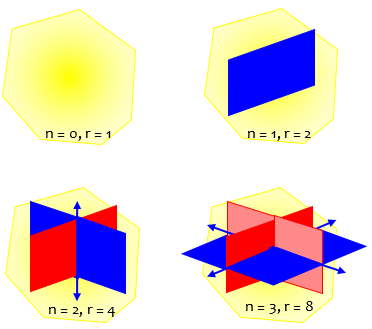Summarizing:

Let me list all the results above:

number of points, lines, planes:
0  1  2  3  4  5  6  7
dim    --------------------------
1     1  2  3  4  5  6  7  8 ...
2     1  2  4  7 11 16 22 29 ...
3     1  2  4  8 15 26 42 64 ...

The first row is obvious - to get any other number in the chart, add together the number to its left to the number above it.

That is, for example, 3 + 4 = 7, and 16 + 26 = 42: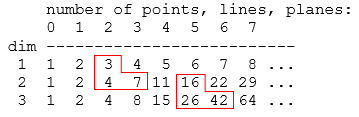### Moving to a formula

You can actually find a formula for it if you like. For dimension 1, it's linear: n+1. For dimension 2, it'll be quadratic, and for dimension 3, cubic. You can find a good reference for finding the formula for dimension 2 at:

http://mathforum.org/dr.math/problems/regimbald3.9.98.html

And by the way, this works fine for hyperspace of still higher dimensions.

The page he referred to is much like one that we saw two weeks ago, for lines in a plane rather than a circle. It turns a difference equation into a sum. Let’s try doing that here.

We saw first that the number of regions in a line cut by n points is $$r(n) = n+1$$ This is the first row in the table.

Then we found that the number of regions in a plane cut by n lines starts with 1, then increases sequentially by the number of regions in each new line, cut by as many points as there are lines already; so each number in the second row of the table is 1 plus the sum of numbers to the left in the row above (that is, from 0 through n – 1). So $$r(n) = 1 + \sum_{i=0}^{n-1}(n+1) = 1 + \frac{n(n+1)}{2} = \frac{n^2+n+2}{2}$$ That’s the formula for the second row.

Our goal is the third row. Again, we found that the number of regions in space cut by n planes starts with 1, then increases sequentially by the number of regions in each new plane, cut by as many lines as there are planes already; so each number in the third row of the table is 1 plus the sum of numbers to the left in the row above (that is, from 0 through n – 1). So (using a known formula for the sum of sequential squares) $$r(n) = 1 + \sum_{i=0}^{n-1}\frac{n^2+n+2}{2} = 1+\frac{n(n-1)(2n-1)}{12} + \frac{n(n-1)}{4} + n = \frac{n^3+5n+6}{6}$$

As we did two weeks ago, we could instead have obtained this formula by seeing the table as a difference table, upside-down, which tells us that the formula will be a cubic polynomial, and solving four equations for the four coefficients.

## Why don’t 4 planes make 16 regions?

A similar question was asked again in 2001:

Planes Intersecting Space

A plane divides space into at most two parts.
Two planes divide space into at most four parts.
Three planes divide space into at most eight parts.
Four planes divide space into at most sixteen parts.

Can we say that n planes divide space into at most 2^n parts? Is there a proof of this idea?

Gafur, like many, has fallen into the now-familiar trap of seeing a false pattern; the last number, 16, is wrong.

Doctor Jubal answered this time, starting by showing why 16 doesn’t work:

Hi Gafur,

Thanks for writing to Dr. Math.

Actually, 4 planes cannot divide space into 16 parts, but only 15.  Let's say you have three planes that divide space into 8 parts. They intersect at a single point. Let's say you take a fourth plane that is not parallel to any of the other three, but also passes through the point. It will intersect each of the other three planes in a line, and these three lines will intersect at a single point.

Here are the three planes: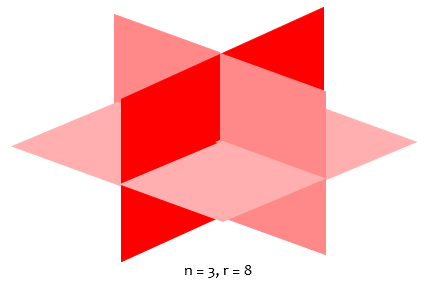Here we see that the fourth plane, cut by three lines, misses two of the 8 regions, so it adds only 6: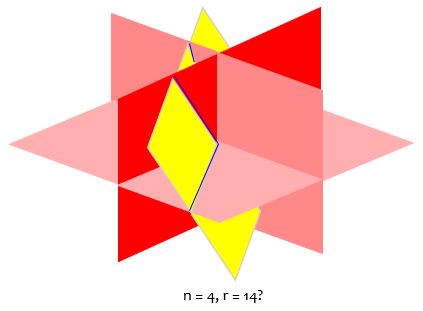That isn’t so easy to see, so we can make it easier:

You can represent this on a sheet of paper by drawing three lines that intersect at a single point. The sheet of paper is the fourth plane.  The three lines are the lines where it intersects the other three.  You will see that the three lines divide the plane into 6 regions.  The first three planes divided space into eight regions. Of these, six of them are divided by the fourth one, if the fourth one passes through the point of intersection of the the other three planes.


Here is our fourth plane: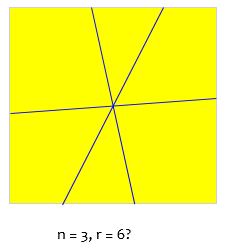With three lines, we’ve (so far) divided this plane into 6 regions; so it must pass through 6 regions of space, adding only 6 to our previous 8 for a total of 14 regions:

Of the other two regions, one is entirely "above" the sheet of paper, and the other is "below" it. Since the fourth plane doesn't have to pass through the point of intersection of the other three planes, it is possible to divide either one of these regions in addition to the original six.

Make a new drawing on your sheet of paper. This time, draw three non-parallel lines that don't intersect in a common point. Instead, each pair of lines intersects each other at one of three different points. You will see they divide the piece of paper into seven regions.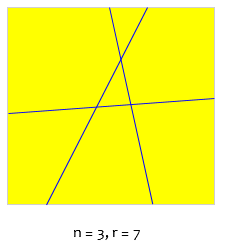This is the case when the fourth plane does not pass through the point of intersection of the other three. It is not parallel to any of the three original planes, so it intersects each of them in a line. Since it does not contain the point of intersection of the other three planes, these three lines do not intersect each other in a single point.

The planes look something like this: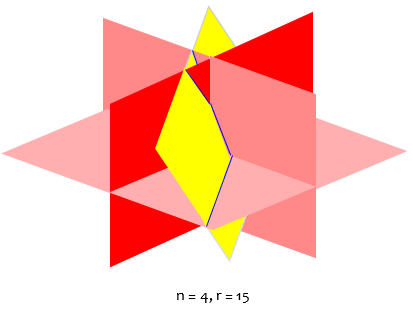But you don’t need to see this to know what’s happening!

You can see that this plane manages to divide seven out of the eight regions the first three planes divide space into. By not passing exactly through the point of intersection, the plane can divide one of the two regions it "missed" in the first case, while still dividing the other six. It is impossible for the plane to divide all eight regions, however, because it is impossible for the plane to pass to both "sides" of the point of intersection of the first three planes.

As a result, four planes can divide space into at most 15 regions.  Three planes divide space into eight regions, and a fourth plane can split all of these regions except one that it must miss because it can only pass to one side or another of the point of intersection of the first three.



In these four planes, there are four points where three planes intersect to make a point. For each one of these points, a fifth plane must pass to one side or the other of it, and so it must miss four out of the 15 regions, and can only split 11 of the 15 into two.  So five planes can only divide space into 15+11 = 26 regions.

The four points represent the 4 ways to choose 3 of the 4 planes: $$\displaystyle{{4}\choose{3}} = 4$$.

### Making a general formula

In general, if you have n planes in space arranged that that no two planes are parallel and no two planes intersect each other in a line that is parallel to any other such line, those n planes will meet at n(n-1)(n-2)/6 points. (This formula comes about because you have n choices of the first plane, n-1 choices the second plane, and n-2 choices of the third plane, but the order of the planes doesn't matter, and there are six ways you could have chosen the same three planes.) At each of these points, the three planes that intersect at that point divide space into eight regions, only seven of which can be split by any single plane. So for each point of intersection of three planes, a new plane must "miss" one regions.

In other words, there are $$\displaystyle{{n}\choose{3}} = \frac{n!}{(n-3)!3!}$$ triples of planes, each of which define an intersection point, and these are assumed to be distinct.

So if n planes divide space into at most r regions, the (n+1)st plane can only intersect r - n(n-1)(n-2)/6 of them, so n+1 planes can divide space into at most 2r - n(n-1)(n-2)/6 regions.

Thus, 4 planes can divide space into 15 regions, 5 planes can divide space into 26 regions, 6 planes into 42 regions, 7 planes into 64 regions, 8 planes into 93 regions, 9 planes into 130 regions, 10 planes into 176 regions, and so on.

This is again a recursive formula; if we rewrite it to take n planes rather than n + 1, we get $$r(n) = 2r(n-1) – \frac{(n-1)(n-2)(n-3)}{6}$$ I won’t try to turn this into an explicit formula, since we’ve already seen one; but we can at least check it against previously verified numbers. For n = 7, we get $$r(7) = 2r(6) – \frac{(6)(5)(4)}{6}= 2(42)-20 = 64$$ which is correct.

## In search of formulas …

Looking elsewhere, I have seen a number of interesting formulas for this. The most interesting, perhaps, is this: $$r(n) = {n\choose 0}+{n\choose 1}+{n\choose 2}+{n\choose 3}$$ This amounts to the number of spaces (1), plus the number of planes (n), plus the number of lines of intersection (pairs of planes), plus the number of points of intersection (triples of planes), counting up by dimension. And in fact, $${n\choose 0}+{n\choose 1}+{n\choose 2}+{n\choose 3} = 1+n+\frac{n(n-1)}{2}+\frac{n(n-1)(n-2)}{6} = \frac{n^3+5n+6}{6}$$ as we’ve seen before.

Now, we might well wonder whether this beautiful pattern is true in other dimensions.

We’ve already seen that the number of regions into which a line is divided by n points is n + 1; but that is equal to $${n\choose 0}+{n\choose 1} = 1+n$$

We’ve also seen that the number of regions into which a plane is divided by n lines is $$\displaystyle\frac{n^2+n+2}{2}$$; but that is in fact equal to $${n\choose 0}+{n\choose 1}+{n\choose 2} = 1+n+\frac{n(n-1)}{2}$$

All we need is a direct way to get to these formulas. Can you find a way? I’ll leave that as an assignment for the reader. (It’s hidden in what we’ve already gone through!)

### 4 thoughts on “Cutting Up Space Using n Planes”

1.How can we prove that this works in higher dimensions as well? Is it simply due to the fact that any “crossing” of two or more hyperplanes induces another cell? Thus we count every occurrence of these events (n choose 2, n choose 3, up to the dimension). Is this a sufficient proof?

On another note, what if we considered quadratic hyperplanes (i.e. of degree at most 2)? Two parabolas can intersect on at most 4 points (one up one sideways), so could we generalize this to the same formula but with a constant of 4 in front of each binomial coefficient (since each of these “crossings” can happen at most 4 times)?

Thank you and looking forward to hearing from you!

1.I don’t have enough experience with higher dimensions (at least not recently) to be confident of a general statement; but I think this generalization is essentially as simple as you suggest.

I don’t know what you mean by “quadratic hyperplane”, which sounds self-contradictory, and at the least, likely to be much more complicated. If you were to submit a question by our Ask A Question page, you might get a response from another of us with more experience in these areas.

2.Hello, Is there a picture where we are cutting a 3-D space using n=5 planes? The nice picture above is for a 3-D space using n=4. Note, for n=5, r=26.

1.It can be done, but it won’t be easy to count the regions!

I made the picture you refer to by a labor-intensive manual method; but it can be done more easily using GeoGebra. Here is the equivalent image made that way: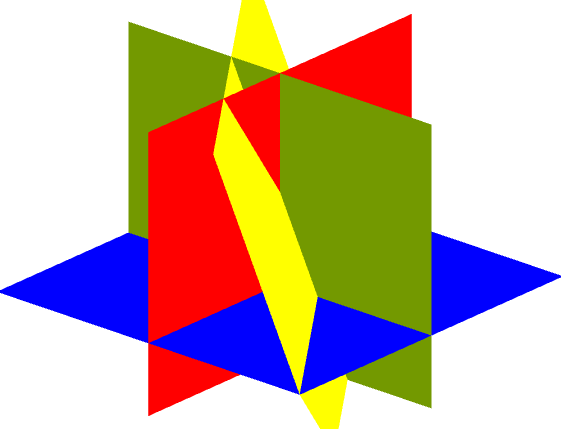Here I’ve added a fifth plane, without taking the time to confirm that all the regions can be somehow recognized in it: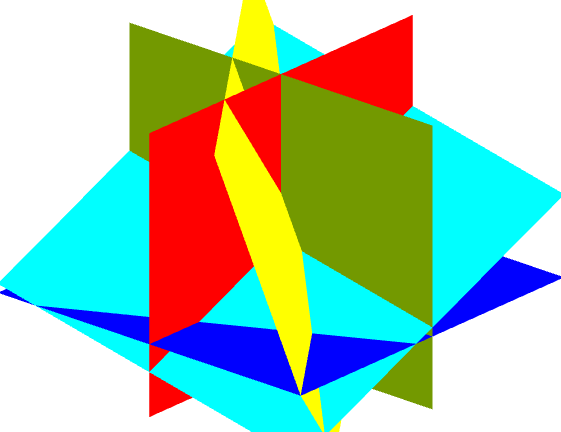Here is a version with transparency: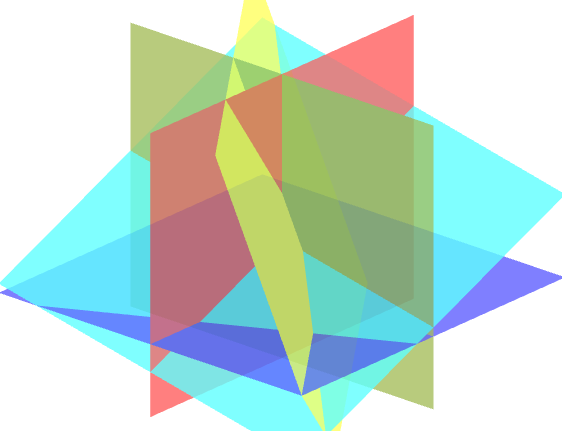This site uses Akismet to reduce spam. Learn how your comment data is processed.